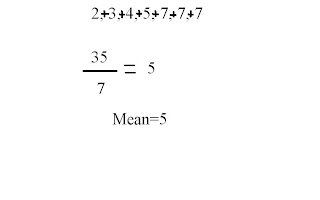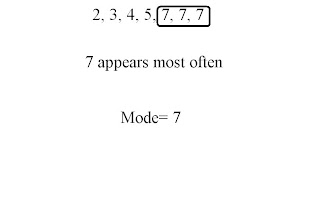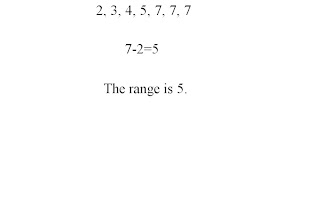### Joseph's Measures of Central Tendancy

Monday, September 29, 2008
• arrange the data in ascending numerical order
• add up all the data

• divide sum by amount of dataMedian:
• arrange the data in ascending numerical order

• cross out or match the same amount of numbers from outside in

• if 2 numbers in the middle then add both together
• divide by 2Mode:
• arrange the data in ascending numerical order

• look and see which number occurs most often
• there could be 0,1,or more modesRange:
• arrange the data in ascending numerical order

• subtract the smallest data from the largestHere is a video on how to do mean, median, mode, and range!!!!!!!mmm